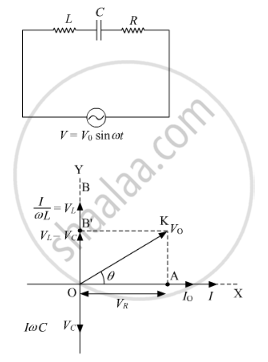# An A.C. Source of Voltage V = V0 Sin ωt is Connected to a Series Combination of L, C and R. Use the Phasor Diagram to Obtain Expression for Impedance of a Circuit and the Phase Angle Between Voltage and Current. - Physics

An a.c. source of voltage V = V0 sin ωt is connected to a series combination of L, C, and R. Use the phasor diagram to obtain the expression for an impedance of a circuit and the phase angle between voltage and current. Find the condition when current will be in phase with the voltage. What is the circuit in this condition called?

#### Solution

i)Voltage of the source is given as

I0→" data-mce-style="position: relative;">V=V0sinωtI0→

Let current of the source be " data-mce-style="position: relative;">I=I0sinωt

The maximum voltage across R is vec(V_R)=vec(V_0)R represented along OX.

The maximum voltage across L is vec(V_L)=vec(I_0) X_Lrepresented along OY and is 90° ahead of vec(I_0)

The maximum voltage across C is vec(V_C)=vec(I_0) X_Crepresented along OC and is lagging behind vec(I_0)by 900

The voltage across L and C has a phase difference of 180°

Hence, reactive voltage isvec(V_L)-vec(V_C)represented by OB

The vector sum ofvec(V_R), vec(V_L) "and "vec(V_C)resultant of OA and OB', represented along OK

OK=V_0=sqrt(OA^2+OB^2)

=>V_0=sqrt(V_R2+(V_L-V_C)^2)=sqrt(I_0R^2+(I_0X-V_C)^2)

=>V_0=I_0sqrt(R^2+(X_L-X_C)^2)

The impedance can be calculated as follows:

Z=V_0/I_0=sqrt(R^2+(X_L-X_C)^2)

When XL  = XC,  the voltage and current are in the same phase. In such a situation, the circuit is known as the non-inductive circuit.

Concept: Power in Ac Circuit: the Power Factor
Is there an error in this question or solution?